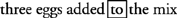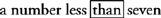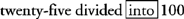## Keywords for Change in Order

Turnaround words indicate a change in order from the original English phrase. Usually, you use keywords in one of two ways:
• Translate the words directly, in the order they are given.
• Recognize leading keywords and find the corresponding AND, TO, BY, or FROM that tell you how to translate the equation.

To help you understand turnaround words, think about the directions on a box of cake mix. If the directions say “three eggs added to the mix,” which do you put into the bowl first? You put the mix into the bowl first, and then add the eggs. The word TO is one of the basic turnaround words discussed in the following section, and to help you remember to turn the expression around, you box the word.## Basic turnaround words

Certain keywords indicate a turn around in the order of the translation. All of the keywords indicating a change in order contain the following words:

• TO
• FROM
• THAN

Addition keywords that indicate a turnaround are

• _____ MORE THAN _____

Example 1: Translate the following: twelve added to negative fourReplace each word with algebraic symbols and turn the expression around.

The expression translates to −4 + 12.

Example 2: Translate the following: add negative three to five

Example 2 uses both a turnaround word and a leading keyword, and it is translated as follows:

1. Because the first word in the expression, ADD, indicates an operation, ADD is a leading keyword. ADD defines the TO, so underline the words before and after TO: “negative three” and “five.”

• add negative three to five

2. Circle the leading keyword and indicate the corresponding TO that it defines; box the turnaround word, TO.3. Translate each underlined expression, replace TO with a plus sign, and turn the expression around.

• The expression translates to 5 + −3.

People sometimes argue that a turnaround word is not necessary with addition because of the Commutative Property of Addition; that is, both −3 + 5 and 5 + −3 result in the same answer for Example 2 (+2) when simplified. However, the latter (5 + −3) shows an understanding of the order indicated by the keyword. You have two reasons to learn to translate expressions in the correct order:

• Good habits are formed for correct translation of subtraction and division expressions, which are not commutative.
• Just as the makers of a cake mix intend for you to put the cake mix in the bowl first and add the eggs later, the author of the problem intends to have you perform the addition in the prescribed order.

## Subtraction turnaround words

Subtraction keywords that indicate a turnaround are

• SUBTRACT _____ FROM _____
• _____ SUBTRACTED FROM _____
• _____ LESS THAN _____

Example 3: Translate the following: a number less than sevenReplace each word with algebraic symbols and turn the expression around.

• The expression translates to 7 − x.

Example 4: Translate the following: subtract seventeen from fifty‐four

1. Because the word SUBTRACT is a leading keyword that defines FROM, underline the words before and after FROM: “seventeen” and “fifty‐four.”

• subtract seventeen from fifty‐four

2. Circle the leading keyword and indicate the corresponding FROM that it defines; box the turnaround word, FROM.3. Translate each underlined expression, replace FROM with a minus sign, and turn the expression around.

• The expression translates to 54 − 17.

## Multiplication turnaround words

None of the multiplication keywords indicates a turnaround. All multiplication expressions can be translated using the direct translation strategy or leading keywords.

The product of a number and 8 can be translated n × 8, but most often, you see the expression written as 8 × n or 8 n, because mathematicians have set a standard that the coefficient is written before the variable. (In this example, the number 8 is the coefficient.)

Note: The expression 8 n uses implied multiplication. Multiplication is implied when a number is placed next to a variable, or when a number is placed next to an expression surrounded by parentheses. Although a multiplication sign is not shown, its use is implied.

## Division turnaround words

Division keywords that indicate a turnaround are:

• DIVIDE _____ INTO _____
• _____ DIVIDED INTO _____

Notice that TO, a basic turnaround word, is included in the word INTO and indicates a turnaround.

Example 5: Translate the following: divide five into 125

Solve Example 5 as follows:

1. Because the word DIVIDE is a leading keyword that defines INTO, underline the words before and after INTO: “five” and “125.”

• divide five into 125

2. Circle the leading keyword and indicate the corresponding INTO that it defines; box the turnaround word, INTO.3. Translate each underlined expression, replace INTO with a division sign, and turn the expression around.

• The expression translates to 125 ÷ 5.

Example 6: Translate the following: twenty‐five divided into one hundred